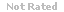Loading... Please wait...

(TCO 1) Which one is non-volatile?

Price:
\$14.99

Product Description

1. (TCO 1) Which one is non-volatile?
2. (TCO 1) Which one has RAS and CAS pins?
3. (TCO 1) With a 24-bit address bus, we get _____ bytes of memory space.
4. (TCO 2) Which one dictates how large of data can be carried into CPU?
5. (TCO 2) The four blocks in a diagram of a general computer system are
6. (TCO 2) Given the following table for the simple CPU of Lecture 1, write the assembly-language code to ADD the contents of Locations 20, 21, and 22. Then, place the result into Location 23.
7. (TCO 1) Which one is non-volatile?
8. (TCO 1) Which one uses capacitor for memory cell?
9. (TCO 1) A given CPU has a 19-bit address bus. How much memory space does it have?
10. (TCO 2) Which one is unidirectional?
11. (TCO 2) The function of the CPU is to
12. (TCO 2) Given the following table for the simple CPU of Lecture 1,
13. (TCO 1) Which one is non-volatile?
14. (TCO 1) Which one uses a one transistor for memory cell?
15. (TCO 1) With a 24-bit address bus, we get _____ bytes of memory space.
16. (TCO 2) Which one dictates how large of data can be carried into CPU?
17. (TCO 2) Operations performed by the ALU include
18. (TCO 2) Given the following table for the simple CPU of Lecture 1,
19. (TCO 1) Flash memory is:
20. (TCO 1) Which one has RAS and CAS pins?
21. (TCO 1) With a 24-bit address bus, we get _____ bytes of memory space.
22. (TCO 2) What direction does data flow on a READ bus cycle?
23. (TCO 2) The function of the CPU is to
24. (TCO 2) Given the following table for the simple CPU of Lecture 1, wr
25. (TCO 1) A given CPU has a 19-bit address bus. How much memory space does it have?
26.
ite the assembly-language code to ADD the contents of Locations 20, 21, and 22. Then, place the result into Location 23.
27. (TCO 1) Which one is used for boot-up when the power is turned on?
28. (TCO 1) Which one uses capacitor for memory cell?
29. (TCO 1) A given CPU has a 19-bit address bus. How much memory space does it have?
30. (TCO 2) What direction does data flow on a WRITE bus cycle?
31. (TCO 2) The function of the CPU is to
32. (TCO 2) Given the following table for the simple CPU of Lecture 1,
33. (TCO 1) Which one is used for boot-up when the power is turned on?
34. (TCO 1) Which one needs refreshing?
35. (TCO 1) A given CPU has an 18-bit address bus. How much memory space does it have?
36. (TCO 2) Which one defines the amount of memory space that a CPU can support (for a byte-addressable CPU)?
37. (TCO 2) Operations performed by the Control Unit include
38. (TCO 2) Given the following table for the simple CPU of Lecture 1,
39. (TCO 1) Which one is volatile?
40. (TCO 1) Which one uses a one transistor for memory cell?
41. (TCO 2) Which one dictates how large of data can be carried into CPU?
42. (TCO 2) Which of the following is sequential logic (a clocked state machine)?
43. (TCO 2) Given the following table for the simple CPU of Lecture 1, there are three numbers stored at Locations 20, 21, and 22. Let’s name these locations X, Y, and Z. Let’s name Location 23, RESULT. Write the assembly-language code to perform the equation:  RESULT = X + Y – Z;

Customers Who Viewed This Product Also Viewed

• \$24.99
• \$15.00
• \$8.99
• \$17.99
• \$9.99

• \$14.99